## Nineteen coding problems for beginners & kids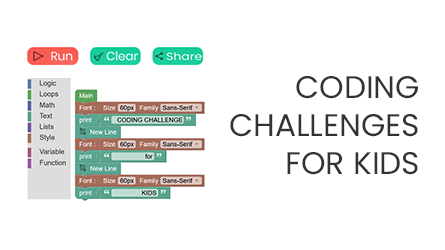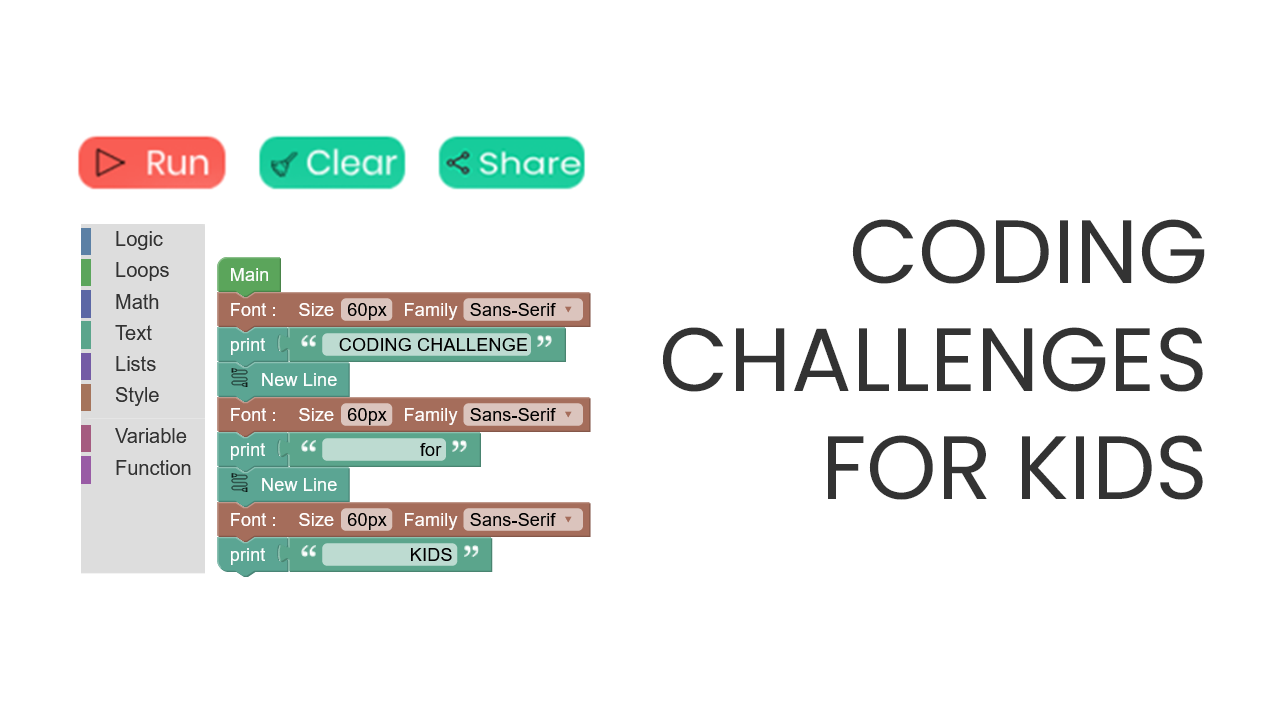Coding improves maths, reasoning, logical & thinking skills, starting at any stage.

Following are the nineteen coding problems for beginners and kids. These challenges are designed in such a way that kids can understand & execute using any coding gaming console, while the beginners can take these as learning challenges and code them using C, C++ or Java language.

Let’s start with some basic, easy programming problems for beginners & kids.

Challenge 1

DefinitionChallenge 2

Definition
You have three components in your birthdate. Date, Month and Year. Do a total of these 3 components and print the answer along with label “Date total:”.
For example if your date of birth is 30th of January 2020 (Which is Havi.co’s birthdate 🙂
Then add 30, 1 and 2020. The answer will be 2051. Print this in following format.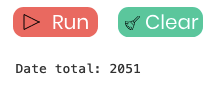Constraint
You need to do the sum within the program itself. Don’t do the total outside and bring the number in the code.

Challenge 3
Print the numbers 1 to 20

Definition
Print the number 1 to 20, each to be in a new line like following.Constraint
You can use the print command only once.

Challenge 4
Take date as user input and then return the total as output

Definition
So this challenge refers back to challenge 2 where you did a total of all three components of the birth date. You entered the components within the program however.

This time, let’s edit the program in a way so it asks the user to input the 3 components i.e. date, month and year.

You can do the total of the birth date components entered by the user.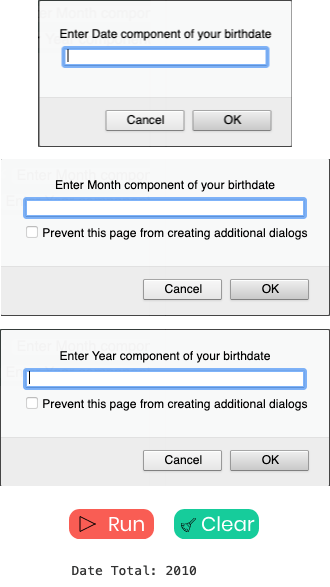Challenge 5
Hours to minutes

Definition
In this challenge we will ask the user the enter the number of hours. For example they enter 3.

The program should convert the hours to minutes and display as output.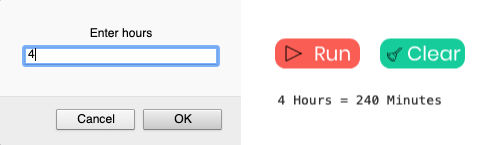Challenge 6
Validate the date

Definition
This challenge is an alteration of challenge 4. You asked user to enter the date components in that.

What if a user enters 35 as date or 18 into month? That’s not valid, right? So you need to check and validate the values entered by the user. Process the date total only if user has entered valid values into each date component.

Product should also consider the number of days into each month while validating.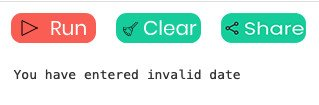Challenge 7
Designing Mario Pyramid

Definition
You must have seen this Pyramid pattern if you have played the Mario game.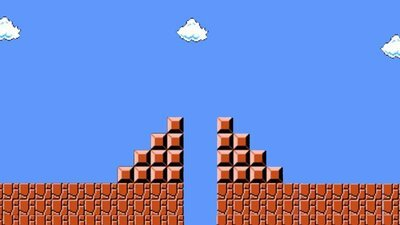You can first try preparing the pattern, first on the graph/grid paper. Place the dots at appropriate locations. That will help you prepare the algorithm well.

And then prepare the mario pattern.Pattern programs for kids & beginners
Challenge 8
Your weight (Mass, scientifically) on different planets and the sun.

Definition
Are you of 41 KG? That’s on the earth. If you go out on a different planet you weight will change, without even loosing them. Following is the matrix of relative surface gravity of all the planets. Relative means they are relative to earth. All you need to do is multiply your weight with gravity figure of the particular planet and you get your weight on that planet.And then write a program that asks user for their weight and then displays their weight on different planets and the sun.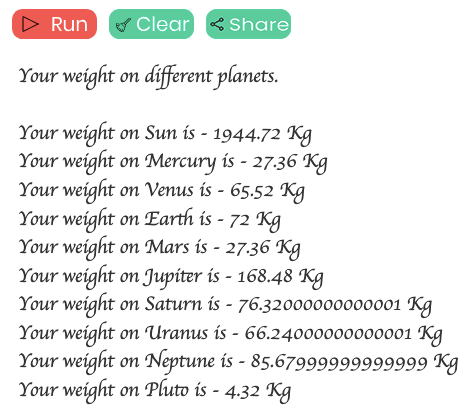Challenge 9
Calculator

Definition
Prepare a program for a basic calculator

1) Ask user for the operation they want to perform out of + – * and /. You can add more operations later.2) Ask for two numbers to perform the operation on.
3) Display the answer along with the equation.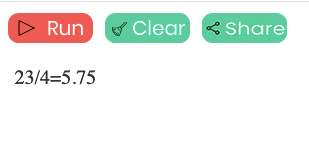Hint:
Use if else ladder and a function too.

Challenge 10
Reverse the number

Definition
Write a program that reverses the number entered by the user.

In this program you will first ask user to enter the number. Store the number into a variable and then reverse the number and print as output.Hint: You will have to use round down, reminder and repeat while commands to solve this problem.

We have prepared a worksheet which will help you understanding the math behind reversing a number. Try this and you will be better able to perform the program then.

Challenge 11
Print odd numbers between two numbers

Definition
In this program you will first ask user to enter two numbers, the start range and end range. Then print all Odd (and even in a separate program) numbers between these two numbers.

Each number should be printed in a new line and can use only one print command for this.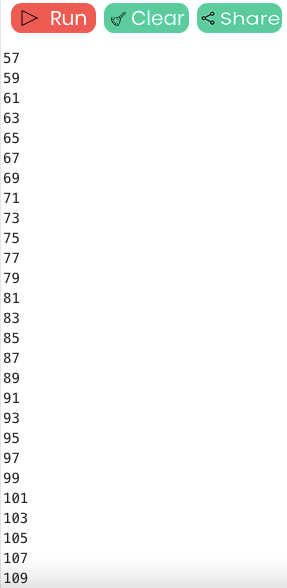Challenge 12

Find average of given numbers

Definition

Ask user to enter how many numbers they want to average out. And then ask the same count of numbers.

Find average of the entered numbers and show the output.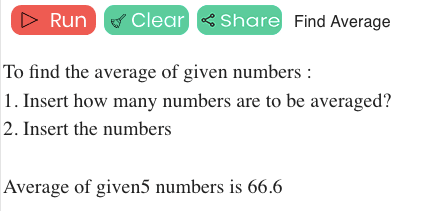We have prepared a worksheet which will help you understanding the math behind averaging the numbers. Try this and you will be better able to perform the program then.

Challenge 13
Check if the number is even or odd

Definition
Ask the user to enter a number and then check if the given number is even or odd.

Display the result in output.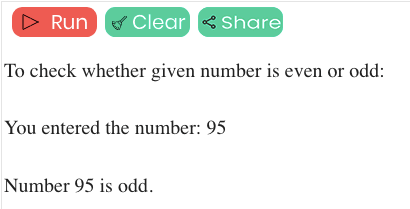Challenge 14
Check if the number is positive or negative

Definition
Ask the user to enter a number and then check if the given number is positive, negative or zero.

Display the result in output.Challenge 15
Check if the number is prime or not

Definition
Ask the user to enter a number and then check if the given number is prime number or not (a composite number).

A number which can not be divided by any other number except 1 and number itself, is called prime number. For example 19 can not be divided by any numbers other than 1 and 19. Hence 19 is a prime number.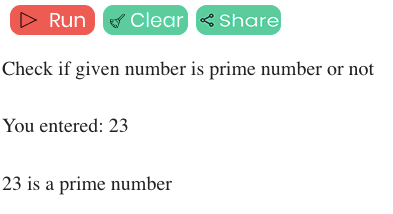Challenge 16
Find the percentage out of given marks

Definition
Ask the user to enter the number of subjects and then ask marks obtained in each of those subjects. Assume eahch the subjects were of 100 marks, can you find the percentage one has got?Challenge 17
Check if the year is a leap year

Definition
Ask the user to enter the year and check if the year is a leap year.

A leap year is the one which can be divided by 4 without and remainder.Challenge 18
Print Fibonacci series

Definition
The Fibonacci series presents the numbers where each number is a sum of 2 immediate previous numbers.

This program should ask user for a number, say X and the program will then print first X numbers of Fibonacci series.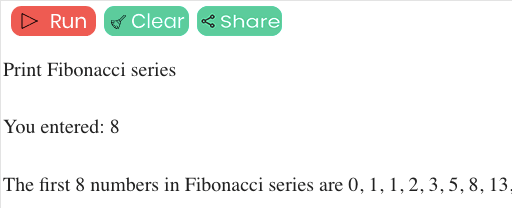Challenge 19
Calculate factorial

Definition
Calculate the factorial of the number entered by the user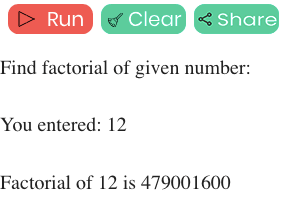Comment down your experience with the programming challenges for kids and beginners.

### Havi Camp

[time_change], 6 PM IST, on zoom call.

A class of Technology and future skills for age 5 to 15.

Get an invite